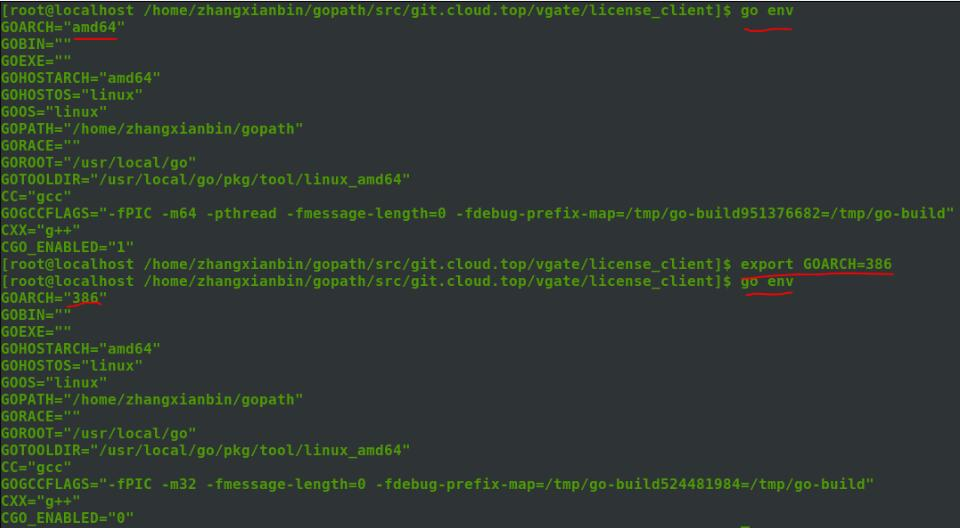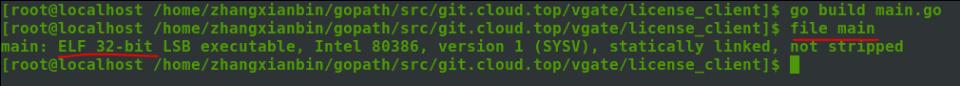# golang 64位linux环境下编译出32位程序操作

1.执行 go env 查看当前go环境配置

2.执行 export GOARCH=386 配置go输出系统平台为32位

3.go build 编译程序

4.使用file main 指令查看编译出的可执行程序是否为32位import ( 'encoding/binary' 'math') func Float32ToByte(float float32) []byte { bits := math.Float32bits(float) bytes := make([]byte, 4) binary.LittleEndian.PutUint32(bytes, bits) return bytes} func ByteToFloat32(bytes []byte) float32 { bits := binary.LittleEndian.Uint32(bytes) return math.Float32frombits(bits)} func Float64ToByte(float float64) []byte { bits := math.Float64bits(float) bytes := make([]byte, 8) binary.LittleEndian.PutUint64(bytes, bits) return bytes} func ByteToFloat64(bytes []byte) float64 { bits := binary.LittleEndian.Uint64(bytes) return math.Float64frombits(bits)}